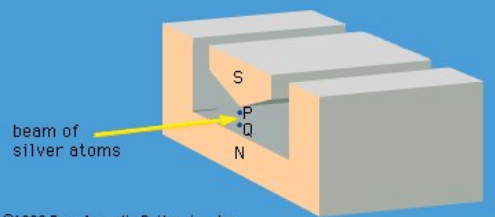Incompatible Observables

# Incompatible Observables - Notes | Study Modern Physics for IIT JAM - Physics

 1 Crore+ students have signed up on EduRev. Have you?

The measurement of two observables with different sets of state functions is a quite different situation. Measurement of one observable gives a certain result. The state function after the measurement is, as always, one of the states of that observable; however, it is not a state function for the second observable. Measuring the second observable disturbs the system, and the state of the system is no longer one of the states of the first observable. In general, measuring the first observable again does not produce the same result as the first time. To sum up, both quantities cannot be known at the same time, and the two observables are said to be incompatible.
A specific example of this behaviour is the measurement of the component of angular momentum along two mutually perpendicular directions. The Stern-Gerlach experiment mentioned above involved measuring the angular momentum of a silver atom in the ground state. In reconstructing this experiment, a beam of silver atoms is passed between the poles of a magnet. The poles are shaped so that the magnetic field varies greatly in strength over a very small distance (Figure 2). The apparatus determines the ms quantum number, which can be +1/2 or −1/2. No other values are obtained. Thus in this case the observable has only two states—i.e., N = 2. The inhomogeneous magnetic field produces a force on the silver atoms in a direction that depends on the spin state of the atoms. The result is shown schematically . A beam of silver atoms is passed through magnet A. The atoms in the state with ms = +1/2 are deflected upward and emerge as beam 1, while those with ms = −1/2 are deflected downward and emerge as beam 2. If the direction of the magnetic field is the x-axis, the apparatus measures Sx, which is the x-component of spin angular momentum. The atoms in beam 1 have Sx = +ℏ/2 while those in beam 2 have Sx = −ℏ/2. In a classical picture, these two states represent atoms spinning about the direction of the x-axis with opposite senses of rotation.Figure 2: Magnet in Stern-Gerlach experiment. N and S are the north and south poles of a magnet. The knife-edge of S results in a much stronger magnetic field at the point P than at Q.

They-component of spin angular momentum Sy also can have only the values +ℏ/2 and −ℏ/2; however, the two states of Sy are not the same as for Sx. In fact, each of the states of Sx is an equal mixture of the states for Sy, and conversely. Again, the two Sy states may be pictured as representing atoms with opposite senses of rotation about the y-axis. These classical pictures of quantum states are helpful, but only up to a certain point. For example, quantum theory says that each of the states corresponding to spin about the x-axis is a superposition of the two states with spin about the y-axis. There is no way to visualize this; it has absolutely no classical counterpart. One simply has to accept the result as a consequence of the axioms of the theory. Suppose that, the atoms in beam 1 are passed into a second magnet B, which has a magnetic field along the y-axis perpendicular to x. The atoms emerge from B and go in equal numbers through its two output channels. Classical theory says that the two magnets together have measured both the x- and y-components of spin angular momentum and that the atoms in beam 3 have S= +ℏ/2, Sy = +ℏ/2, while those in beam 4 have Sx = +ℏ/2, Sy = −ℏ/2. However, classical theory is wrong, because if beam 3 is put through still another magnet C, with its magnetic field along x, the atoms divide equally into beams 5 and 6 instead of emerging as a single beam 5 (as they would if they had Sx = +ℏ/2). Thus, the correct statement is that the beam entering B has Sx = +ℏ/2 and is composed of an equal mixture of the states Sy = +ℏ/2 and Sy = −ℏ/2—i.e., the x-component of angular momentum is known but the y-component is not. Correspondingly, beam 3 leaving B has Sy = +ℏ/2 and is an equal mixture of the states Sx = +ℏ/2 and Sx = −ℏ/2; the y-component of angular momentum is known but the x-component is not. The information about Sx is lost because of the disturbance caused by magnet B in the measurement of Sy.

The document Incompatible Observables - Notes | Study Modern Physics for IIT JAM - Physics is a part of the Physics Course Modern Physics for IIT JAM.
All you need of Physics at this link: Physics

## Modern Physics for IIT JAM

54 videos|44 docs|15 tests
 Use Code STAYHOME200 and get INR 200 additional OFF

## Modern Physics for IIT JAM

54 videos|44 docs|15 tests

Track your progress, build streaks, highlight & save important lessons and more!

,

,

,

,

,

,

,

,

,

,

,

,

,

,

,

,

,

,

,

,

,

;